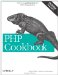# Recipe 4.8. Turning an Array into a String

#### 4.8.1. Problem

You have an array, and you want to convert it into a nicely formatted string.

#### 4.8.2. Solution

Use join( ):

`// make a comma delimited list \$string = join(',', \$array);`

Or loop yourself:

`\$string = ''; foreach (\$array as \$key => \$value) {     \$string .= ",\$value"; } \$string = substr(\$string, 1); // remove leading ","`

#### 4.8.3. Discussion

If you can use join( ), do; it's faster than any PHP-based loop. However, join( ) isn't very flexible. First, it places a delimiter only between elements, not around them. To wrap elements inside HTML bold tags and separate them with commas, do this:

`\$left  = '<b>'; \$right = '</b>'; \$html = \$left . join("\$right,\$left", \$html) . \$right;`

Second, join( ) doesn't allow you to discriminate against values. If you want to include a subset of entries, you need to loop yourself:

`\$string = ''; foreach (\$fields as \$key => \$value) {     // don't include password     if ('password' != \$key) {         \$string .= ",<b>\$value</b>";     } } \$string = substr(\$string, 1); // remove leading ","`

Notice that a separator is always added to each value and then stripped off outside the loop. While it's somewhat wasteful to add something that will be subtracted later, it's far cleaner and efficient (in most cases) than attempting to embed logic inside of the loop. To wit:

`\$string = ''; foreach (\$fields as \$key => \$value) {     // don't include password     if ('password' != \$value) {         if (!empty(\$string)) { \$string .= ','; }         \$string .= "<b>\$value</b>";     } }`

Now you have to check \$string every time you append a value. That's worse than the simple substr( ) call. Also, prepend the delimiter (in this case a comma) instead of appending it because it's faster to shorten a string from the front than the rear.

Recipe 4.9 for printing an array with commas; documentation on join( ) at http://www.php.net/join and substr( ) at http://www.php.net/substr.PHP Cookbook: Solutions and Examples for PHP Programmers
ISBN: 0596101015
EAN: 2147483647
Year: 2006
Pages: 445

Similar book on Amazon